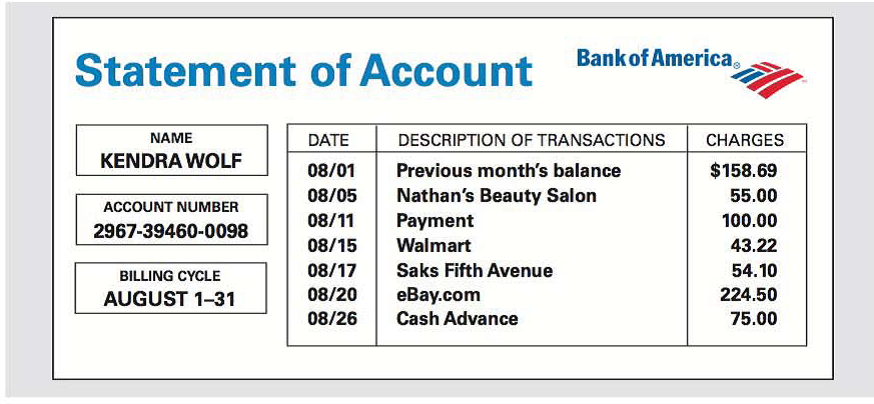Chapter 13.I, Problem 2TIE### Contemporary Mathematics for Busin...

8th Edition
Robert Brechner + 1 other
ISBN: 9781305585447

#### Solutions

Chapter
Section### Contemporary Mathematics for Busin...

8th Edition
Robert Brechner + 1 other
ISBN: 9781305585447
Textbook Problem

# Kendra Wolf has a Bank of America revolving credit account with an 18% annual percentage rate. The finance charge is calculated by using the average daily balance method. The billing date is the first day of each month, and the billing cycle is the number of days in that month. During the month of August. Kendra’s account showed the following activity.What is the finance charge for August, and what is Kendra.s new balance?

To determine

To calculate: The finance charge and new balance if Kendra Wolf has a Bank of America account with the previous month balance is 158.69, purchases and cash advances is $451.82, payment and advances is$100 and the annual percentage rate is 18%.

Explanation

Given Information:

Kendra Wolf has a Bank of America account with the previous month balance is 158.69, purchases and cash advances is $451.82, payment and advances is$100 and the annual percentage rate is 18%.

The table showing the transactions is as follows:

 Dates Activity Amount ($) 08/01 Previous month’s balance 158.69 08/05 Nathan’s beauty salon 55.0 08/11 Payment 100.00 08/15 Walmart 43.22 08/17 Saks fifth avenue 54.10 08/20 eBAy.com 224.50 08/26 Cash advance 75.00 Formula used: Follow the steps to determine finance charge and new balance through unpaid balance method: Step 1: Monthly periodic rate is computed by dividing the annual percentage rate by the number of months in a year. Periodic Rate=Annual Percentage Rate12 Step 2: Average daily balance is computed by dividing the total sum of daily balances by the number of days in a billing cycle. Average daily balance=Sum of daily balancesdays in billing cycle Step 3: Finance charge can be computed by multiplying the average daily balance with the periodic rate. Finance Charge=Average daily balance×periodic rate Step 4: New balance is computed by adding all previous month balance, finance charge, purchases and cash advances and subtracts the total of payments and credits. New balance= Previous rate +Finance rate+Purchases and cash advancesPayments and credits. Calculation: The formula for the periodic rate is computed by dividing the annual percentage rate by the number of months in a year. Substitute the annual percentage rate by 18% and time period by 12 months. Periodic Rate=Annual Percentage Rate12=18%12=1.5% Hence, the periodic rate is 1.5%. Consider that previous balance is 158.69, annual percentage rate is 18%. The table showing the transactions is as follows:  Dates No. of days Activity/ Amount Unpaid balance (amount+charge−payment) ($) Daily balances (unpaid balance×days) (\$) August 1−4 4 Previous month’s balance 158

### Still sussing out bartleby?

Check out a sample textbook solution.

See a sample solution

#### The Solution to Your Study Problems

Bartleby provides explanations to thousands of textbook problems written by our experts, many with advanced degrees!

Get Started

#### 37. If find

Mathematical Applications for the Management, Life, and Social Sciences

#### True or False: div curl F = 0.

Study Guide for Stewart's Multivariable Calculus, 8th

#### Simplify each expression. log773

College Algebra (MindTap Course List)